×

Search anything:

# Hash Map / Hash table

#### Data Structures hash map HashingGet this book -> Problems on Array: For Interviews and Competitive Programming

Hash map is a widely used efficient data structure that used to store data which can be searched in constant time O(1). It is also referred as hash table, unordered map, dictionary, hash set and others. This data structure is implemented over an array that maps keys to values. Hence, hash map can be seen as a set of key value pairs. Each key is a number in the range of 0 to the array size – 1, generated by a hash function.

A good example for hash map is phone book. A phone book has names and phone numbers. In this case, the names are the keys, the phone numbers are the values. Imagine having a billion integers, using a search algorithm like linear search will take months while **hash map will take a second.

### Idea

The idea behind hash map is simple yet very effective.

Imagine an array of size N. Array A will have indexes from 0 to N-1 and we can access data at index i (0 <= i <= N-1) instantly that is in constant time O(1).

Note that in other data structures like linked list, accessing data at a particular index takes linear time O(N).

Idea 1: Use Array as a underlying data structure for Hash map

Idea 2: Use index of the array as the data

At this point, the problem is that we need to accept any data into a hash map such as strings, user defined objects and others and array accept indexes from 0 to N-1. For this, we need to convert any given data to our permissible range that is o to N-1. This process is known as hashing and will be performed by a function/ algorithm known as hash function.

Idea 3: Use array data as a pair of the index data

Since, we have stored data as the index, we have space for values. To utilize this, we will store data as pairs. In case, this pair is avoided, hash map is known as hash set.

### Overview

There are four key components in a hash map:

• a hash function: to map a data to an integer index
• collision handling: how to handle two data points that map to the same index
• put: how to add data to a hash map? in O(1) time
• get: how to retrieve data from a hash map? in O(1) time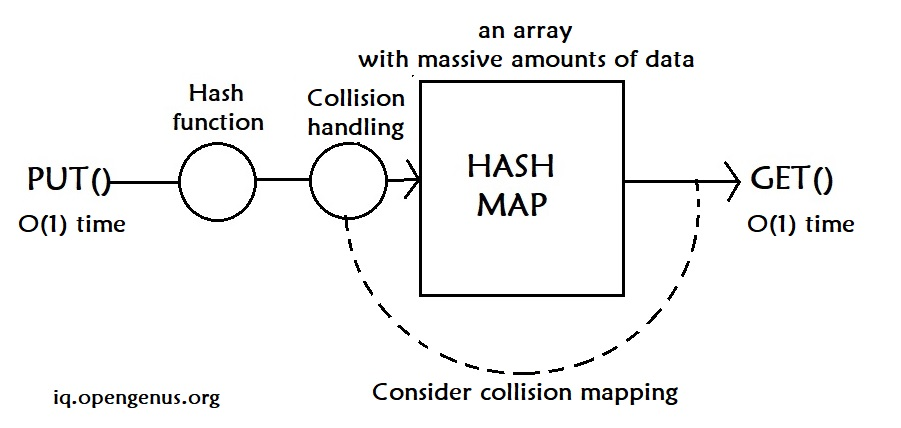### Hash Function

A hash function is used to generate the index (hash code) of the key in the array. Ideally, each index a hash function generates should be unique, but in practice, it is extremely difficult.

The default hash function might not satisfy the programmer's needs. For example, in Java, a hash code is generated based on the object's address. If we need to compare other characteristics about two objects, like two people's name and age, we need to write a new hash function that generates hash code based on name and age. Note that when overriding the hash code function, we should always override the equal function too, because that is the method which checks is two objects are the same or not.

``````    public class Peach {
private double weight;
private String color;
// In this example, we will compare two peaches,
// if they have the same color, same weight,
// then we say they are equal to each other

public Peach(double weight, String color) {
this.weight = weight;
this.color = color;
}

@Override
public boolean equals(Object obj) {
if (this == obj)
return true;
if (obj == null)
return false;
if (this.getClass() != obj.getClass())
return false;
Peach peach = (Peach) obj;
return weight == peach.weight && color.equals(peach.color);
}

@Override
public int hashCode() {
int hash = 37;
hash = hash + (int) weight;
hash = hash + (color == null? 0 : color.hashCode());
return hash;
}

public static void main(String[] args) {
Peach peach1 = new Peach(10, "Red");
Peach peach2 = new Peach(10, "Red");
System.out.println(peach1.hashCode());
System.out.println(peach1.equals(peach2));
System.out.println(peach2.hashCode());
}

}
``````

Properties:

1. A hash function always returns a number
2. Two equal objects (based on the equal() method) always have the same hash code
3. Two different objects don't always have the same hash code

Here is the procedure of storing objects in the array using a hash function: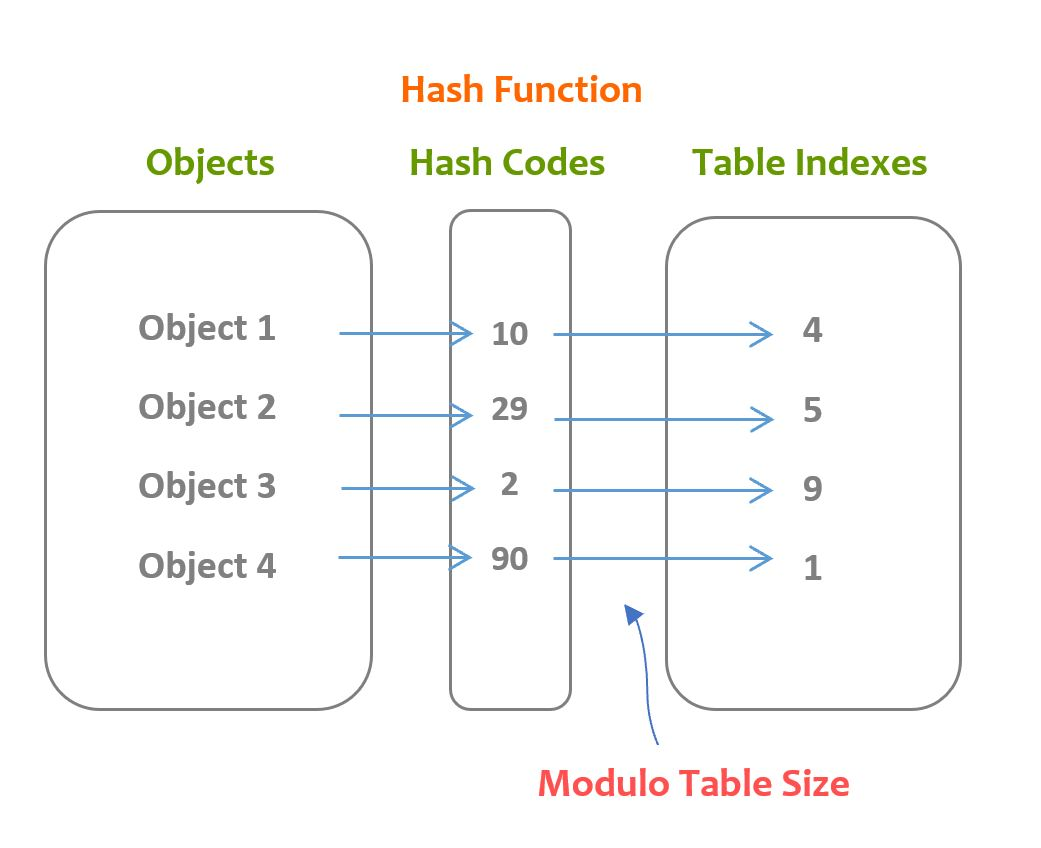Here is a hash table example: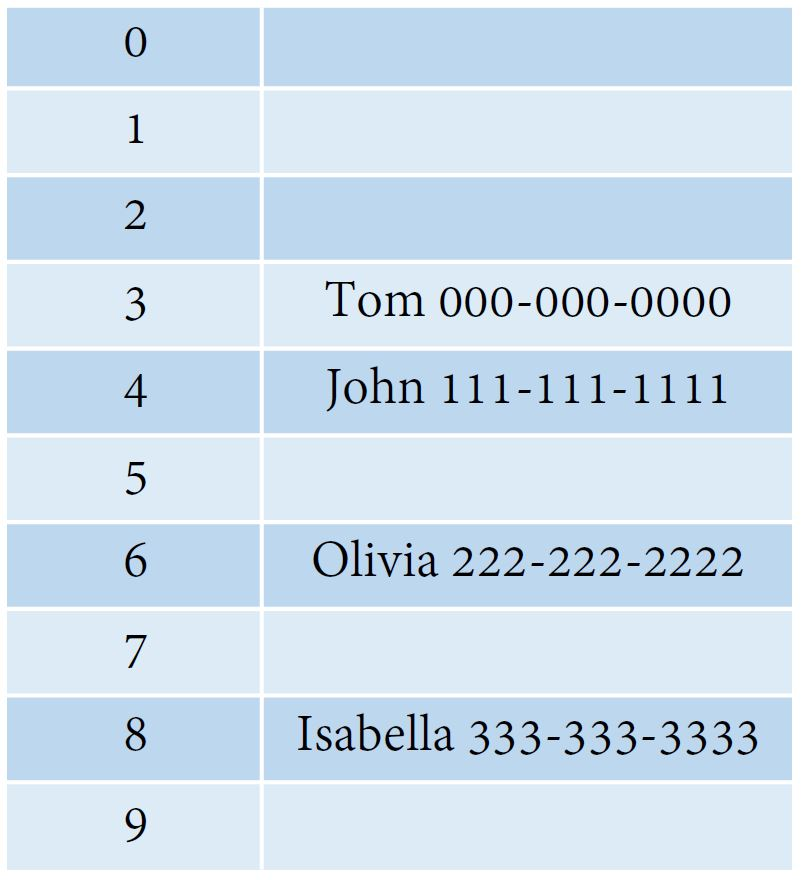Here we use a hash function which generates indexes based on the name length. So, Tom will be in index 3, John will be in index 4. But if there was a Mia which also has a length of 3, a collision will happen.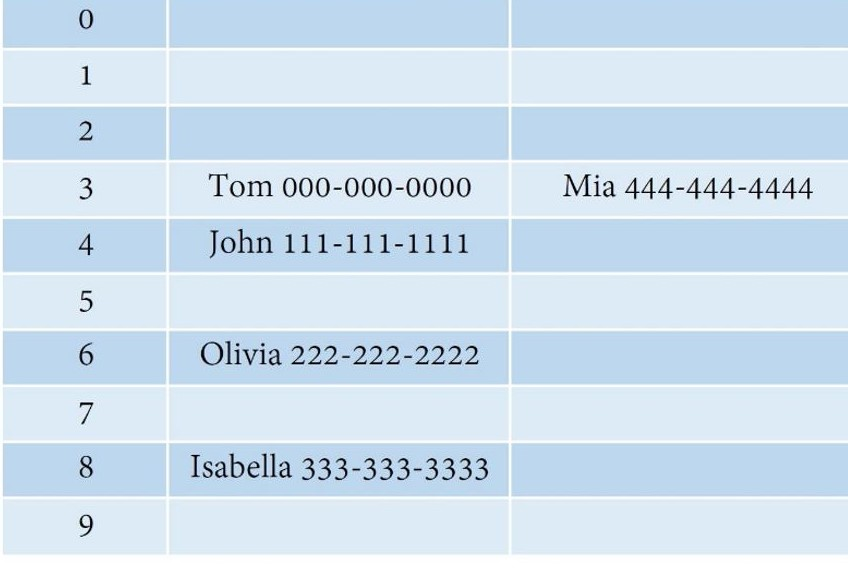### Collision & handling

Collision happens when different objects in the hash map are mapped to the same location.

#### 1. Separate Chaining

How it works: put all the objects which have the same hash code into a linked list (the hash map is then an array of lists).

To perform a search: we need to determine which list to traverse by using the hash function, and then search in that linked list.

To perform insert: depending on if duplicates are accepted or not, we check the target list to see if the element we want to insert already exists or not. We then insert the element at the front of the list because first, it is convenient; second, the recently inserted elements are more likely to be needed in the future.

#### Linear Probing

How it works: when there is collision, this method looks for the next available space and insert the object there.

For example, instead of putting Mia and Tom in a linked list, linear probing finds the next space available to insert object Mia.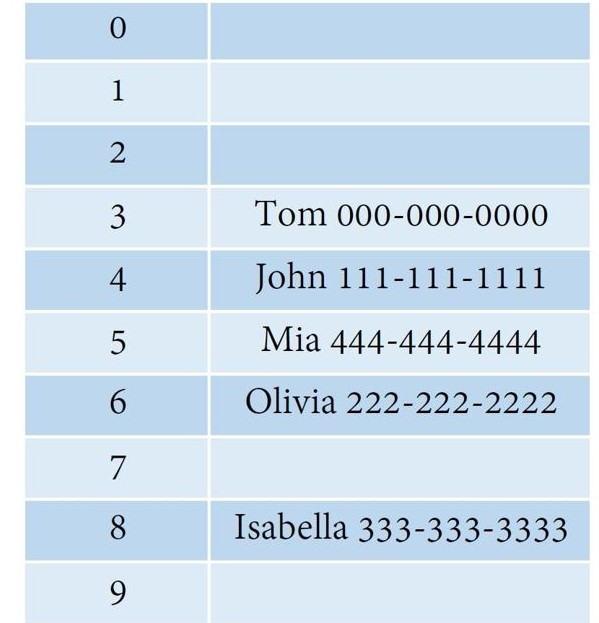In this process, primary clustering, which means the slots after to the hash position are being filled up, can happen. For example, if the primary hash index is x, the probing will go x+1, x+2, x+3 and so on. This can reduce the performance.

How it works: it is a collision handling method that eliminates the primary clustering problem. Like what the name itself, this method uses a quadratic collision function. A common choice is f(i) = i^2, i stands for index.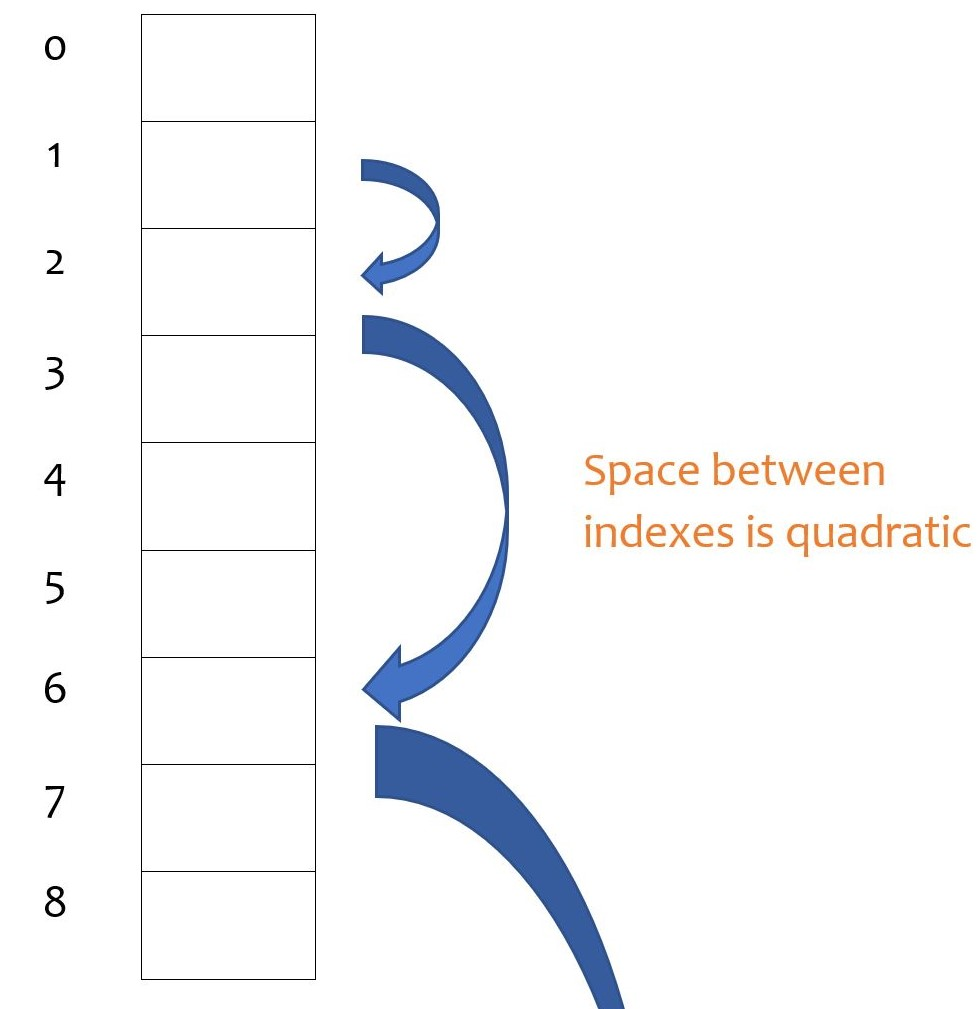In this process, secondary clustering can happen. Just like primary clustering, the slots after the hash position can be filled up, but created by quadratic probing. For example, if the primary hash index is x, the probing will go x+2, x+4, x+9 and so on. Secondary clustering is less problematic for the performance.

#### Double Hashing

How it works: this method requires a second hash function to resolve collision. When a collision happens, this method uses the second hash function to generate a new hash code for the object. A common choice is hash(key) = R - (key % R), R stands for a prime smaller than the table size.

Two key things:

1. the function must never evaluate to 0
2. try to make sure that all the cells can be probed

### Rehashing

How it works: when the hash map is more than 50% full, rehashing technique creates a new hash map which has the first prime that is roughly twice as large as the original map as its size, and then hash each object to the new map.Rehashing is an expensive operation because every object has to be moved around.

### Operations & Pseudocode

• the following uses separate chaining to avoid collisions

Time Complexity for put() and get()
Average: O(1)
Worst: O(n)

Hash code allows us to go to the desired index directly instead of iterating through the array. If the hash function is well-written, we only need to look at the linked list at that index. And there should be reasonable amount of objects in one linked list since the objects are evenly distributed in the array with a good hash function. So the average time complexity should be O(1).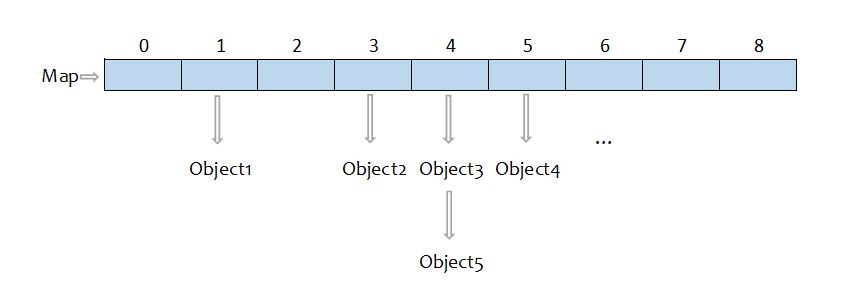However, if the hash function is poorly-written, in other words, objects are not evenly distributed, the linked list will be long and takes a long time to iterate. In this case, the time complexity is O(n).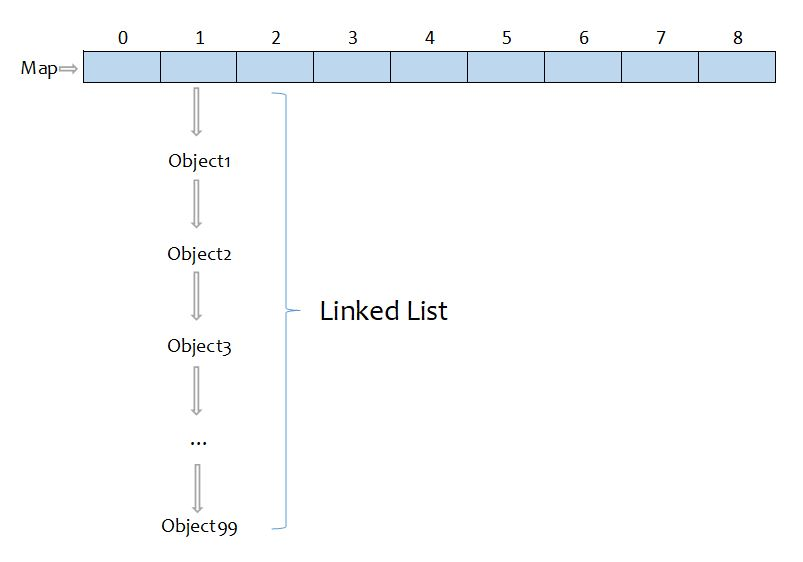### PUT(): insert data into a hash map

put(): put the key-value pair to the map

``````    Check if the key is null:
if true:
place the key at table
return
else:
calculate the hash code for this key
Use the hash code to generate an index for the key
Check all the keys in the linked list at that index
replace the old value with new value, return
else:
append the new key-value pair in the linked list, return

return
``````

### GET(): get data from a hash map

``````get(): takes the key and returns the associated value

Check if the key is null:
if true: return the value at table
else:
calculate the hash code for this key
find out the index of this key
iterate through the linked list at that index,
check if the key exists
if true: return the value
else: return null
``````

### Implementation examples

``````    import java.util.*;

public class HashTableExample {
public static void main(String[] args) {
Map<String, Long> map = new HashMap<>();

map.put("Olivia", 2222222222L);
map.put("John", 1111111111L);
map.put("Cat", 9999999999L);
map.put("Dog", 5555555555L);
// Note: if you put more than certain amount of objects in a hash map
// it automatically expands itself

System.out.println("Olivia's number: " + map.get("Olivia"));
System.out.println("John's number: " + map.get("John"));

System.out.println("Returns true if key Olivia exists: " + map.containsKey("Olivia"));
System.out.println("Returns true if value 2222222222 exists: " + map.containsValue(2222222222L));

map.remove("John"); // Remove key John and its value

map.replace("Olivia", 1234567890L); // Replace Olivia's number with something else

// The following shows how to iterate through a map with iterator and keyset()
Iterator iterator = map.keySet().iterator();
System.out.print("These are the keys contained in this map: ");
while (iterator.hasNext()){
String key = (String) iterator.next();
System.out.print(key + " ");
}

Set set = map.entrySet(); // entrySet() returns the mappings in this map
System.out.println("\nThis is the mappings in this map: " + set);

}
}
``````

### Applications

1. Searching any data from a massive data set in O(1) time complexity
2. Compilers use hash map to record the declared variables
3. Online spelling check
4. Caches in both software (caches in a browser) and hardware (memory caches)
5. Routers#### Yao Yao

Software Developer at City of Seattle | Associate of Science (2016 to 2019) in Computer Science at Seattle Central College

Hash Map / Hash table